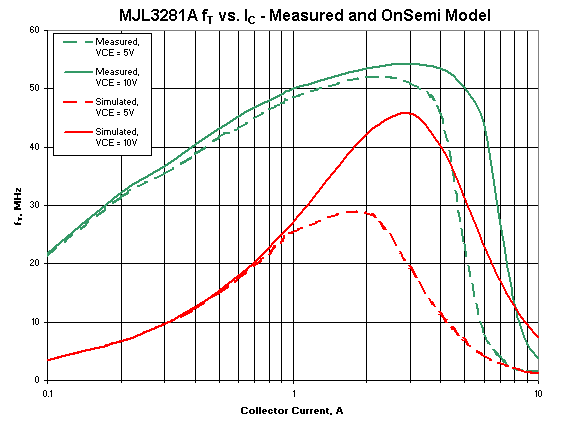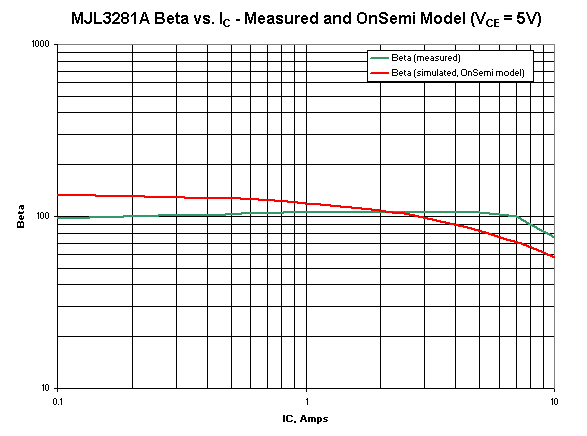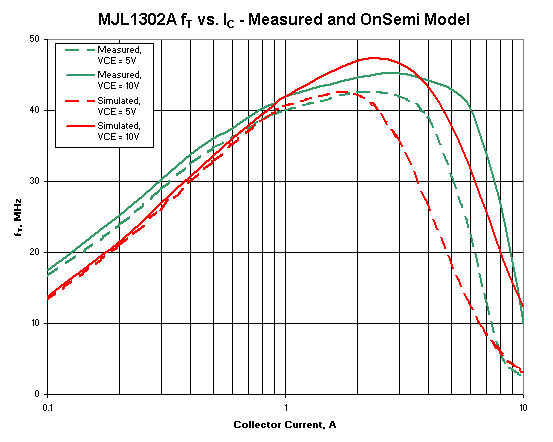# Section 2: Comparison Between Measured Data and Factory-Provided Models

## Measured vs. Simulated Data - MJL3281A

### fT vs. IC

Below are the MJL3281A measured data of fT vs. IC and the corresponding data predicted by the SPICE model. The measured data are shown in green and the simulated data are shown in red. The solid lines are for VCE = 10 V and the dashed lines are for VCE = 5 V.Figure 2. Measured and Simulated Data of fT vs. IC for MJL3281A

At an IC of 100 mA (a typical bias current for the device), the simulated fT is 3.45 MHz, while the measured fT is about 21 MHz. For small-signal analysis, the simulated bandwidth of an emitter follower will therefore be low by about a factor of 6. Also, as VCE is reduced from 10 V to 5 V, the simulation shows a greater reduction of fT than the measurement does. Clearly there is much room for improvement in the ability of the models to accurately predict fT vs. current.

### Cob and Cib vs. Reverse Voltage

Below in Figure 3 are the plots of simulated and measured data of Cob and Cib vs. reverse voltage for the MJL3281A. Measured data are again shown in green and simulated data are shown in red. The solid lines represent Cib while the dotted lines represent Cob. The simulated data were not obtained directly from the simulator. Instead, they were obtained by substituting the model parameter values into the junction capacitance formulas given in Massobrio and Antognetti. The capacitance formulas are described in detail later in the section on parameter extraction, together with the model parameters they use. However, it's worth noting that one important parameter of the formulas is the extrapolated Cib value at VCB = 0. This parameter has a significant effect on the simulated fT vs. collector current in the low-current region. Therefore, if the simulated values of Cib vs. VCB deviate significantly from the measured values, there will be a corresponding error in the simulated values of fT vs. IC in the low-current region. This turns out to be the root cause of the discrepancy between measured and simulated fT for the MJL3281A in the low-current region.

It can be seen that the simulated data for Cib are much larger than the measured data. This explains the discrepancy between the measured and simulated low-current values of fT. It is also seen that the simulated data for Cob are lower than the measured data. However, the effect of Cob on fT is minimal, so the error in Cib dominates the fT error.

### β vs. Collector Current

Below in Figure 4 are plots of β vs. collector current comparing the measured datasheet values with the simulated values obtained from the OnSemi model with VCE = 5V. The green trace is measured data and the red trace is simulated data.Figure 4. Measured and Simulated Data for MJL3281A β vs. IC

It is seen that the simulated β vs. collector current shows a pronounced reduction with increasing collector current that's not present in the actual data. One excellent feature of this device is its nearly constant β from low currents all the way up to 7 A. As with the data for fT vs. collector current, simulations will show performance worse than actual devices.

## Measured vs. Simulated Data - MJL1302A

### fT vs. IC

Below in Figure 5 are the MJL1302A measured data of fT vs. IC and the corresponding data predicted by the SPICE model. As for the MJL3281A, the measured data are shown in green and the simulated data are shown in red. The solid lines are for VCE = 10 V and the dashed lines are for VCE = 5 V.Figure 5. Measured and Simulated Data of fT vs. IC for MJL1302A

Correlation of measured and simulated data is much better than with the MJL3281A, but could still be improved.

### Cob and Cib vs. Reverse Voltage

Below in Figure 6 are the plots of simulated and measured data of Cob and Cib vs. reverse voltage for the MJL1302A. Measured data are again shown in green and simulated data are shown in red. The solid lines represent Cib while the dotted lines represent Cob. It is seen that the correlation between measured and simulated capacitances is nearly perfect. As mentioned in the discussion of the corresponding data for the MJL3281A, having accurate simulated Cib vs. reverse voltage is essential to getting accurate data of fT vs. current in the low-current region. But despite the almost perfect matching of measured and simulated Cib vs. reverse voltage, the error in fT at low currents is still not negligible. In the parameter extraction section, it will be shown that fine-tuning of the capacitance parameters of the model can result in significant improvements in the accuracy of fT vs. current in the low-current region without sacrificing accuracy in the simulated data of Cib vs. reverse voltage.